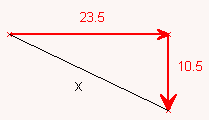SEARCH HOMEMath Central Quandaries & QueriesQuestion from Nancy, a parent: A child runs due east for 23.5m and then she runs 10.5m due south. How far is she from her starting point?Hi Nancy.

Let me draw this as a diagram:This is a right triangle, so you can use Pythagoras' Theorem to solve it:

x2 = 23.52 + 10.52

Solve for x.

Cheers,
Stephen La Rocque.Math Central is supported by the University of Regina and The Pacific Institute for the Mathematical Sciences.